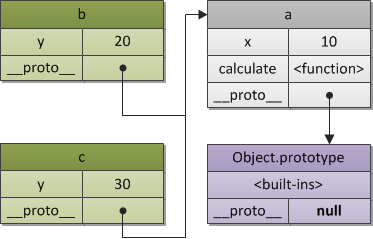## JavaScript 基于原型的继承

|     |   0 浏览

• 基于类（class-based）
• 基于原型（prototype-based）
• 基于元类（metaclass-based）

JavaScript 采用的是基于原型的实现方案，这也是 JavaScript 最重要的语言特性之一。

ES6之前，在 JavaScript 中没有类（class）的概念，对象实例是通过构造函数来创建的。构造函数中有个 `prototype`属性，该属性的值就是原型。

## 空的对象

``````1Object.prototype.__proto__;  // null
``````

`Object.prototype`是一个“空”的对象，“空”的对象只有预定义的属性（包括方法）。这些属性会被所有的对象继承，使用下面的语句可以查看这些预定义的属性。

`````` 1Object.getOwnPropertyNames(Object.prototype).forEach(function log(element, index, array) {
2  console.log('[' + index + '] = ' + element);
3});
4 = constructor
5 = __defineGetter__
6 = __defineSetter__
7 = hasOwnProperty
8 = __lookupGetter__
9 = __lookupSetter__
10 = isPrototypeOf
11 = propertyIsEnumerable
12 = toString
13 = valueOf
14 = __proto__
15 = toLocaleString
``````

## 原型链

`__proto__ `主要是安放在一个实际的对象中，用它来产生一个原型链，用于寻找方法名或属性，等等。

`````` 1var a = {
2x: 10,
3calculate: function (z) {
4return this.x + this.y + z;
5}
6};
7
8var b = {
9y: 20,
10__proto__: a
11};
12
13var c = {
14y: 30,
15__proto__: a
16};
17
18// call the inherited method
19b.calculate(30); // 60
20c.calculate(40); // 80
````````````1var b = Object.create(a, {y: {value: 20}});
2var c = Object.create(a, {y: {value: 30}});
``````